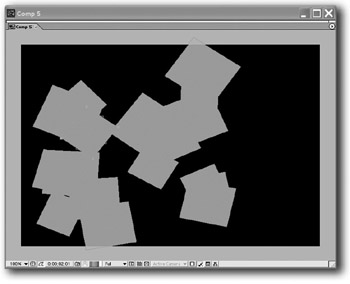# Useful Expressions III

Random Motion by Dan Ebberts

These are the basic expressions for generating random motion. These expressions independently generate random values for position, scale, rotation, and opacity and move to the new values at a random speed.

The expressions can easily be adapted for other properties and effects, 1D or 3D motion, etc.

### This is the expression for position:

` segMin = .3; //minimum segment duration segMax = .7; //maximum segment duration minVal = [0.1*thisComp.width, 0.1*thisComp.height]; maxVal = [0.9*thisComp.width, 0.9*thisComp.height]; end = 0; j = 0; while ( time >= end){  j ++;  seedRandom(j,true);  start = end;  end += random(segMin,segMax); } endVal = random(minVal,maxVal); seedRandom(j-1,true); dummy = random(); //this is a throw-away value startVal = random(minVal,maxVal); ease(time,start,end,startVal,endVal) Adjust segMin and segMax to set the range of times it will take to move from one position to the next. `### This is the expression for rotation:

` segMin = .3; //minimum segment duration segMax = .7; //maximum segment duration minVal = -720; maxVal = 720; end = 0; j = 0; while ( time >= end){  j ++;  seedRandom(j,true);  start = end;  end += random(segMin,segMax);  }  endVal = random(minVal,maxVal);  seedRandom(j-1,true);  dummy = random(); //this is a throw-away value  startVal = random(minVal,maxVal);  ease(time,start,end,startVal,endVal) `

This is the expression for scale:

` segMin = .3; //minimum segment duration segMax = .7; //maximum segment duration minVal = 50; maxVal = 200; end = 0; j = 0; while ( time >= end){  j ++;  seedRandom(j,true);  start = end;  end  += random(segMin,segMax); } s = random(minVal,maxVal); endVal = [s,s]; seedRandom(j-1,true); dummy = random(); //this is a throw-away value s = random(minVal,maxVal); startVal = [s,s]; ease(time,start,end,startVal,endVal) `

### This is the expression for opacity:

` segMin = .3; //minimum segment duration segMax = .7; //maximum segment duration minVal = 10; maxVal = 100; end = 0; j = 0; while ( time >= end){  j ++;  seedRandom(j,true);  start = end;  end += random(segMin,segMax); } endVal = random(minVal,maxVal); seedRandom(j-1,true); dummy = random(); //this is a throw-away value startVal = random(minVal,maxVal); ease(time,start,end,startVal,endVal) `After Effects On the Spot[c] Time-Saving Tips and Shortcuts from the Pros
ISBN: 1578202396
EAN: N/A
Year: 2006
Pages: 447